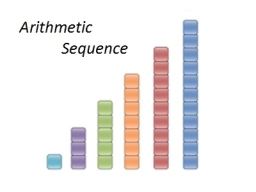# Twice 3

Twice a certain number is added to 10. If the result is minus fourteen. Find the number

x =  -12

### Step-by-step explanation:Did you find an error or inaccuracy? Feel free to write us. Thank you!

Tips for related online calculators
Do you have a linear equation or system of equations and looking for its solution? Or do you have a quadratic equation?